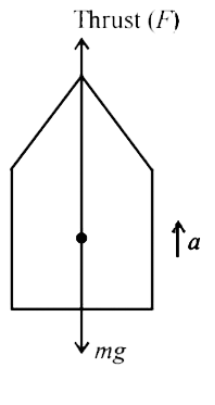Question

# A rocket with a lift-off mass $3.5×{10}^{4}kg$ is blasted upwards with an initial acceleration of $10m/{s}^{2}$. The initial thrust of the blast is:

Open in App
Solution

## Given dataThe mass of the rocket is $m=3.5×{10}^{4}kg$Acceleration of blast is $a=10m/{s}^{2}$Rocket thrustRocket thrust is a reaction force on the rocket due to the outflow of rocket fuel at a very high speed.Due to this thrust rocket moves in space.DiagramCalculation of initial rocket thrustNow, the net force exerted on the rocket is $F={F}_{t}-mg$, where, ${F}_{t}$ is the upward thrust on the rocket.Now, the initial thrust is ${F}_{t}=F+mg=ma+mg\phantom{\rule{0ex}{0ex}}or{F}_{t}=m\left(a+g\right)=\left(3.5×{10}^{4}\right)×\left(9.8+10\right)\phantom{\rule{0ex}{0ex}}or{F}_{t}=\left(3.5×{10}^{4}\right)×19.8\phantom{\rule{0ex}{0ex}}or{F}_{t}=6.9×{10}^{5}N.$Therefore, The initial thrust of the blast is $6.9×{10}^{5}N$Suggest Corrections0Similar questionsExplore more